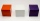# Cube + expression of a variable from the formula - math problems

#### Number of problems found: 88

• Water containerThe cube-shaped container is filled to two-thirds of its height. If we pour 18 liters, it will be filled to three-fifths of the height. What is the volume of the whole container?
• A plasticineJožko modeled from plasticine. He used 27g of plasticine to model a 3 cm long cube. How many grams of plasticine will it need to mold cubes with an edge of 6cm?
• Length of the edgeFind the length of the edge of a cube with a cm2 surface and a volume in cm3 expressed by the same number.
• Water levelHow many cm will the water level in a cube-shaped tank with an edge of 3 m drop if we discharge 189 hl of water?
• Wooden containerThe locksmith should cover the cube-shaped wooden container with a metal sheet inside. The outer edge of the container is 54cm. The wall thickness is 25 mm. The container has no lid. Calculate. How many sheets will be needed to cover it?
• Two cubesThe surfaces of two cubes, one of which has an edge of 22 cm longer than the second are differ by 19272 cm2. Calculate the edge length of both cubes.
• Cube surface and volumeThe surface of the cube is 500 cm2, how much cm3 will be its volume?
• Calculate 3Calculate the cube volume whose edge is 3x-1,3x-1,3x-1
• The volume 2The volume of a cube is 27 cubic meters. Find the height of the cube.
• Area to volumeIf the surface area of a cube is 486, find its volume.
• CubeThe sum of all cube edges is 30cm. Find the surface area of the cube.
• Cube surface and volumeFind the surface of the cube with a volume of 27 dm3.
• Edges or sidesCalculate the cube volume, if the sum of the lengths of all sides is 276 cm.
• Minimum surfaceFind the length, breadth, and height of the cuboid-shaped box with a minimum surface area, into which 50 cuboid-shaped blocks, each with length, breadth, and height equal to 4 cm, 3 cm, and 2 cm respectively, can be packed.
• Cube wallsFind the volume and the surface area of the cube if the area of one of its walls is 40 cm2.
• Cube into sphereThe cube has brushed a sphere as large as possible. Determine how much percent the waste was.
• Cube edgesThe sum of the lengths of the cube edges is 42 cm. Calculate the surface of the cube.
• Surface of cubesPeter molded a cuboid 2 cm, 4cm, 9cm of plasticine. Then the plasticine split into two parts in a ratio 1:8. From each piece made a cube. In what ratio are the surfaces of these cubes?
• Cube edgesIf the edge length of the cube increases by 50%, how does the volume of this cube increase?
• Cuboid edges in ratioCuboid edges lengths are in ratio 2:4:6. Calculate their lengths if you know that the cuboid volume is 24576 cm3.

Do you have an exciting math question or word problem that you can't solve? Ask a question or post a math problem, and we can try to solve it.

We will send a solution to your e-mail address. Solved examples are also published here. Please enter the e-mail correctly and check whether you don't have a full mailbox.

Cube Problems. Expression of a variable from the formula - math problems.